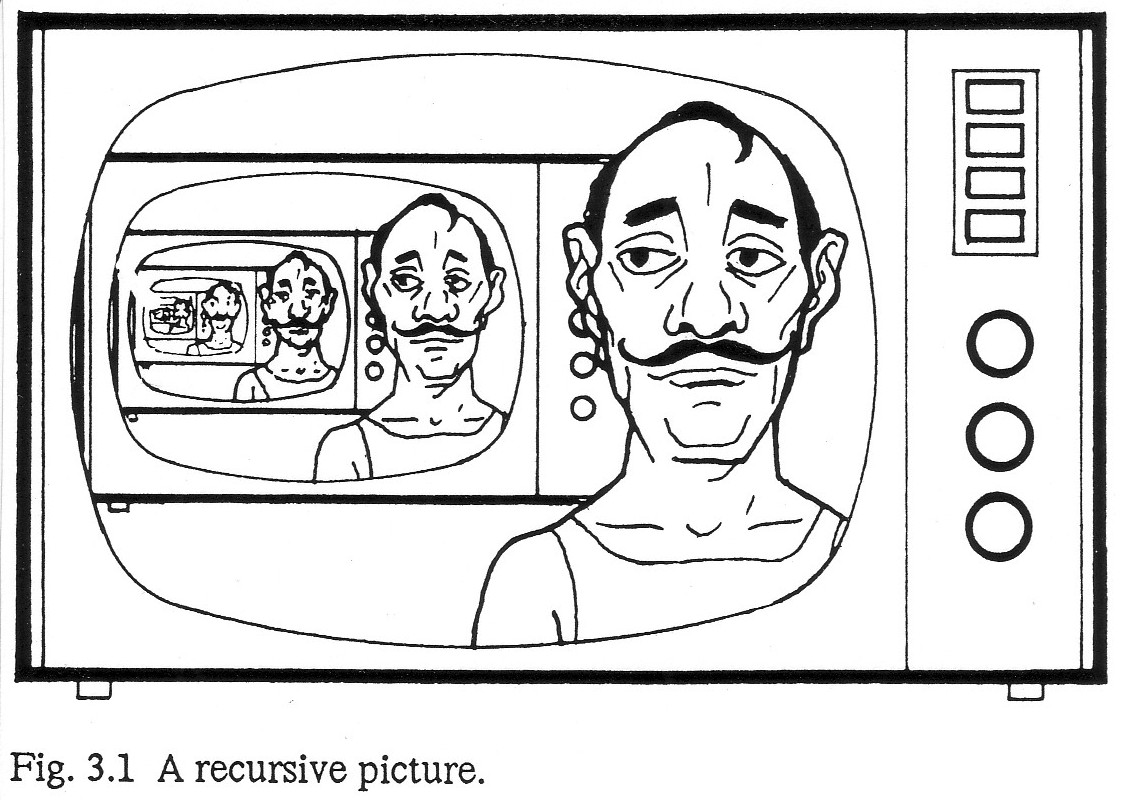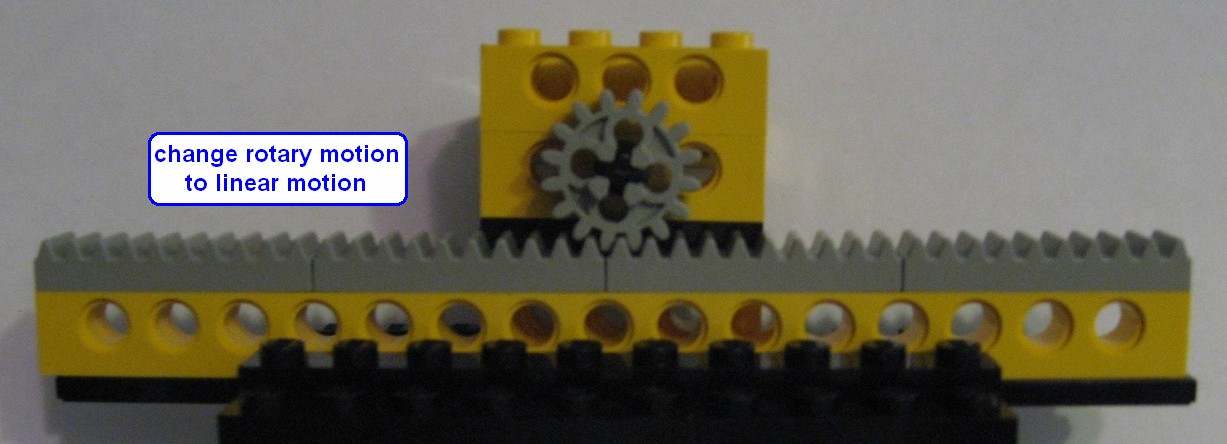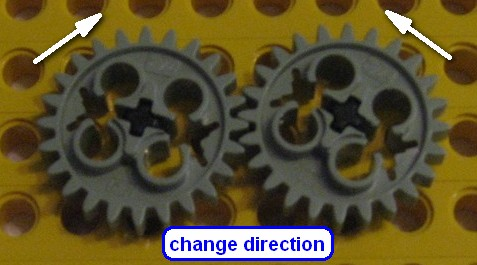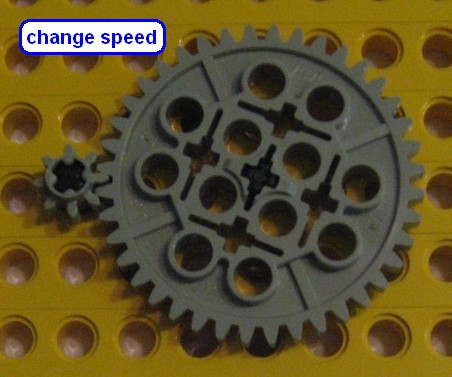# A pilgrimage of algorithms

 Engineering is the art of not constructing; or, to define it rudely but not inaptly, it is the art of doing that well with one dollar which any bungler can do with two. [Arthur Wellington]Opportunities: problem solving, algorithm versus data structure versus brute force, engineering versus bludgeoning, "additional levels of indirection", time versus space optimization, recursion, C++ STL container classes, container class copy semantics, struct versus pointer-to-struct

At work we needed a "pretty print" utility to take the "flat" output of Unix's "ps" (list processes) command, and present it hierarchically. I found this domain to be very small, and yet very intriguing. Each iteration produced more inspiration than closure. I decided to document several of the algorithms that resulted; with the idea that you can never have too many examples in a Computer Science program for use as demos, case studies, or assignments.

Here is sample output of "ps -ef". The second column is the process' "pid". The third column is the process' parent's pid. Everything after the time column is the command line that initiated the process (with a sequence number artificially prepended).

```dvbop    05230 00001  0 21:33 ?        00:00:00 01 /dvbnfs/wms/current/bin/wmsd -w /dvbnfs/wms/cur
dvbop    05233 05230  0 21:33 ?        00:00:00 02 /dvbnfs/wms/current/bin/LRM -w /dvbnfs/wms/curre
root     11474 04237  0 22:59 pts/1    00:00:00 03 su - dvbop
dvbop    11513 11474  0 22:59 pts/1    00:00:00 04 -csh
dvbop    20513 27351  3 23:42 ?        00:00:00 05 /usr/bin/perl /ramdisk/system/bc//bc_
dvbop    20524 20513  0 23:42 ?        00:00:00 06 sh -c /ramdisk/system/bc//packetChar -
dvbop    20527 20513  0 23:42 ?        00:00:00 07 sh -c /ramdisk/system/bc//packetChar -c
dvbop    20529 20513  0 23:42 ?        00:00:00 08 sh -c /ramdisk/system/bc//packetChar -cl
dvbop    20531 20513  0 23:42 ?        00:00:00 09 sh -c /ramdisk/system/bc//packetChar -cli
dvbop    20533 20524 92 23:42 ?        00:00:00 10 /ramdisk/system/bc//packetChar -clist 2009
dvbop    20534 20527 91 23:42 ?        00:00:00 11 /ramdisk/system/bc//packetChar -clist 20090
dvbop    20535 20529 91 23:42 ?        00:00:00 12 /ramdisk/system/bc//packetChar -clist 200907
dvbop    20536 20531 90 23:42 ?        00:00:00 13 /ramdisk/system/bc//packetChar -clist 2009072
dvbop    20541 27351  3 23:42 ?        00:00:00 14 /usr/bin/perl /ramdisk/system/bc//bc_sdw_algo_
dvbop    20542 11513  0 23:42 pts/1    00:00:00 15 ps -ef
dvbop    20543 11513  0 23:42 pts/1    00:00:00 16 grep dvbop
dvbop    20544 11513  0 23:42 pts/1    00:00:00 17 sort -k 2n
dvbop    20545 20541  0 23:42 ?        00:00:00 18 sh -c /ramdisk/system/bc//fft_sbtc_mt -t 4 2009
dvbop    27336 05233  0 22:41 ?        00:00:00 19 NOS      -i nos.ini -s NOS
dvbop    27341 05233 40 22:41 ?        00:24:22 20 DIF      -i sw_sbt.ini -s DIF
dvbop    27351 05233  2 22:41 ?        00:01:27 21 FFT_SBT  -i sw_sbt.ini -s FFT_SBT
root     31082 03870  0 22:45 ?        00:00:00 22 sshd: dvbop [priv]
dvbop    31084 31082  0 22:45 ?        00:00:00 23 sshd: dvbop@pts/0
dvbop    31085 31084  0 22:45 pts/0    00:00:00 24 -csh
```
The output needs to demonstrate the hierarchical nature of the data. All processes that do not have a parent in the input should start at the left edge. Children and grandchildren are then indented. The columns of interest are: pid, parent pid, and command line.
```05230--00001--01 /dvbnfs/wms/current/bin/wmsd -w /dvbnfs/wms/cur
05233--05230--02 /dvbnfs/wms/current/bin/LRM -w /dvbnfs/wms/curre
27336--05233--19 NOS      -i nos.ini -s NOS
27341--05233--20 DIF      -i sw_sbt.ini -s DIF
27351--05233--21 FFT_SBT  -i sw_sbt.ini -s FFT_SBT
20513--27351--05 /usr/bin/perl /ramdisk/system/bc//bc_
20524--20513--06 sh -c /ramdisk/system/bc//packetChar -
20533--20524--10 /ramdisk/system/bc//packetChar -clist 2009
20527--20513--07 sh -c /ramdisk/system/bc//packetChar -c
20534--20527--11 /ramdisk/system/bc//packetChar -clist 20090
20529--20513--08 sh -c /ramdisk/system/bc//packetChar -cl
20535--20529--12 /ramdisk/system/bc//packetChar -clist 200907
20531--20513--09 sh -c /ramdisk/system/bc//packetChar -cli
20536--20531--13 /ramdisk/system/bc//packetChar -clist 2009072
20541--27351--14 /usr/bin/perl /ramdisk/system/bc//bc_sdw_algo_
20545--20541--18 sh -c /ramdisk/system/bc//fft_sbtc_mt -t 4 2009
11474--04237--03 su - dvbop
11513--11474--04 -csh
20542--11513--15 ps -ef
20543--11513--16 grep dvbop
20544--11513--17 sort -k 2n
31082--03870--22 sshd: dvbop [priv]
31084--31082--23 sshd: dvbop@pts/0
31085--31084--24 -csh
```
So ... where to begin?

Is this domain sophisticated enough to require data structures and/or algorithms? Is time spent on reflection, abtraction, leverage, and elegance time well spent? Or is code cheap, and anything that delays the production of said code an unwarranted diversion?

Is this a 60 lines-of-code kind of problem, or will it require several hundred LOC?

Do we need to wrestle with "time versus space" (i.e. optimization) issues?

Does a binary tree map well to this domain? Is a more robust B-tree preferable? Is a custom list-of-lists the only approach that will work?

 If you can only think of one possible solution, then you don't fully understand the problem. [source lost]
To populate a hierarchical "tree", what about searching for the top-most processes, then finding each of their children, then finding each of their children, etc?

What about sorting the data first? Is there any kind of "pre-conditioning" of the data that makes the problem easier? [Notice that a couple parent pids are greater than the corresponding child pid. That seems counter-intuitive. You would assume the parent process has a lower pid number, and then each child process would have a higher pid.]

## Brute force design and implementation

Here is a "brute force" design. It started with intuition, and then incrementally evolved as more and more discrepancies in understanding and implementation were identified.
```// contains #includes

struct Node {
string        pid, parent_pid, cmd_line;
vector<Node*> children;
};

// contains create_and_init_node() definition
#include "util_stuff.h"

///////////////////////////////////////////////////////////////////////////////

int main( int argc, char* argv[] ) {
vector<Node>  all_obj;
vector<Node*> all;
string        line;
ifstream      ifs( argv );

// Create a struct per line of input
while (getline( ifs, line ))
all_obj.push_back( create_and_init_node( line ) );

// Create a vector of ptrs to the previous vector of structs
for (int i=0; i < all_obj.size(); ++i)
all.push_back( &(all_obj[i]) );

// Find all the "orphan" processes (no parent in the list)
Node roots;
for (int i=0; i < all.size(); ++i) {
bool found = false;
for (int j=0; j < all.size(); ++j)
if (all[i]->parent_pid == all[j]->pid) {
found = true;
break;
}
if ( ! found)
roots.children.push_back( all[i] );
}

// Populate the hierarchy - iterate through the Nodes, and put each in its parent
for (int i=0; i < all.size(); ++i) {
// First, try to find the Node's parent in the "orphan" list
for (int j=0; j < roots.children.size(); ++j)
if (all[i]->parent_pid == roots.children[j]->pid) {
roots.children[j]->children.push_back( all[i] );
goto break_label;
}
// Else, find the Node's parent in the "all" list
for (int j=0; j < all.size(); ++j)
if (all[i]->parent_pid == all[j]->pid) {
all[j]->children.push_back( all[i] );
break;
}
break_label:;
}

// Report the hierarchy
vector<Node*> parent(10);
vector<int>   child(10);
int level = 0;
int indent = 0;
child[level] = 0;
parent[level] = &roots;
while (level > -1) {
cout << string(indent,' ') << parent[level]->children[ child[level] ]->pid
<< "--" << parent[level]->children[ child[level] ]->parent_pid
<< "--" << parent[level]->children[ child[level] ]->cmd_line << '\n';
// Report children first (depth first)
if (parent[level]->children[ child[level] ]->children.size()) {
indent += 3;
child[level+1] = 0;
parent[level+1] = parent[level]->children[ child[level] ];
level += 1;
// Report siblings next (breadth next)
} else {
child[level] += 1;
// If all sibling have been visited, then return to the parent Node
while (level > -1  &&  child[level] == parent[level]->children.size()) {
level -= 1;
indent -= 3;
child[level] += 1;
}  }  }  }
```
Notice that the "populate the hierarchy" section has a loop within a loop. This has been described as "N squared" behavior, and is routinely viewed as uninspired performance.

The complexity of the "report the hierarchy" section is daunting. This is because of "impedance mismatch" between the domain and our low-level programming mentality. If we thought at a higher level, and used tools like state frames, a stack, and recursion, the code would be greatly simplified. That is the goal of the next iteration.

Notice the nature of the tree-like output. As each Node is visited, it reports its struct members, and then delegates to each of its children. This could be characterized as: suspend, descend, return, and resume. That behavior is a perfect match for the technique known as recursive descent.

Recognizing the opportunity to leverage recursion is an enormous win. Once you get over the abstractness of this tool that is foreign to the senses of mere mortals -- the problem practically solves itself.

 There are two ways of constructing a software design: One way is to make it so simple that there are obviously no deficiencies, and the other way is to make it so complicated that there are no obvious deficiencies. The first method is far more difficult. [C.A.R. Hoare][from Data Structures & Algorithms by Niklaus Wirth]
```#include "header_stuff.h"

struct Node {
string        pid, parent_pid, cmd_line;
vector<Node>  children;
};

// Recursively populate the hierarchy
void add( Node& parent, vector<Node>& nodes ) {
// Find and append all the children of this parent
for (int i=0; i < nodes.size(); ++i)
if (nodes[i].parent_pid == parent.pid)
parent.children.push_back( nodes[i] );
// Then "descend" to each child that has just been added, and continue adding
for (int i=0; i < parent.children.size(); ++i)
}

// Recursively report the hierarchy
void traverse( Node n, int indent ) {
// Report the pids and cmd_line of the Node that was passed to this function
cout << string(indent,' ') << n.pid << "--" << n.parent_pid << "--" << n.cmd_line << '\n';
// Then "descend" to each child and increment the indentation argument
for (int i=0; i < n.children.size(); ++i)
traverse( n.children[i], indent+3 );
}

#include "util_stuff.h"

///////////////////////////////////////////////////////////////////////////////

int main( int argc, char* argv[] ) {
vector<Node> all;
set<string>  pids;
string       line;
ifstream     ifs( argv );
while (getline( ifs, line )) {
all.push_back( create_and_init_node( line ) );
// Collect all the pid strings in a set container
pids.insert( all.back().pid );
}

vector<Node> roots;
for (vector<Node>::iterator it = all.begin(); it != all.end(); ++it)
// If the parent_pid does not exist in the input, then this is an orphan process
if (pids.find( it->parent_pid ) == pids.end()) {
roots.push_back( *it );
// Now that the orphan/root Node has been harvested, remove it from "all"
all.erase( it );
}

for (int i=0; i < roots.size(); ++i)

for (int i=0; i < roots.size(); ++i)
traverse( roots[i], 0 );
}
```

## The magic of indirection

Instead of iterating through the entire "all" vector to populate every Node, there ought to be a way to: go through the list one time, "look up" each parent, and register each child with its parent. The `map` container class provides this powerful "look up" capability.
```#include "header_stuff.h"

struct Node {
string         pid, parent_pid, cmd_line;
vector<Node*>  children;
};

void traverse( Node n, int indent ) {
cout << string(indent,' ') << n.pid << "--" << n.parent_pid << "--" << n.cmd_line << '\n';
for (int i=0; i < n.children.size(); ++i)
traverse( *n.children[i], indent+3 );
}

#include "util_stuff.h"

///////////////////////////////////////////////////////////////////////////////

int main( int argc, char* argv[] ) {
// Use an "associative array" to provide string look-up for each Node
map<string,Node>  all;
string            line;
ifstream          ifs( argv );
while (getline( ifs, line )) {
Node n = create_and_init_node( line );
all[ n.pid ] = n;
}

// Step through each entry and create the "graph" of parent-child relationships
for (map<string,Node>::iterator it = all.begin(); it != all.end(); ++it)
// Guard against trying to dereference a "null" parent
if (all.find( it->second.parent_pid ) != all.end())
// Use the parent_pid field to look-up the parent Node,
//    and append the child Node to the parent's vector
all[ it->second.parent_pid ].children.push_back( &(it->second) );

for (map<string,Node>::iterator it = all.begin(); it != all.end(); ++it)
// Search for the orphan/root Nodes
if (all.find( it->second.parent_pid ) == all.end())
traverse( it->second, 0 );
}
```
The `map` container class provides a "level of indirection" that can be used to implement the illusion of magic in the hands of a craftsman. A physical example of indirection would be gears — which can transmit and change the direction, type, speed, or torque of motion.Compare the "weight" of this design with that of the first design. The savings in size and complexity is striking!

 Measuring software productivity by lines of code is like measuring progress on an airplane by how much it weighs. [Bill Gates]

## Object-oriented version

The previous recursive design requires very little modification to make it "object oriented": the functions have become "instance methods", and the first argument to each function is no longer necessary.

This is a simplified demonstration of the "gang of four" Composite design pattern.

```#include "header_stuff.h"

struct Node {
string        pid, parent_pid, cmd_line;
vector<Node>  children;

void add( vector<Node>& nodes ) {
for (int i=0; i < nodes.size(); ++i)
if (nodes[i].parent_pid == pid)
children.push_back( nodes[i] );
for (int i=0; i < children.size(); ++i)
}
void traverse( int indent ) {
cout << string(indent,' ') << pid << "--" << parent_pid << "--" << cmd_line << '\n';
for (int i=0; i < children.size(); ++i)
children[i].traverse( indent+3 );
}
};

#include "util_stuff.h"

///////////////////////////////////////////////////////////////////////////////

int main( int argc, char* argv[] ) {
vector<Node> all;
set<string>  pids;
string       line;
ifstream     ifs( argv );
while (getline( ifs, line )) {
all.push_back( create_and_init_node( line ) );
pids.insert( all.back().pid );
}

vector<Node>  roots;
for (vector<Node>::iterator it = all.begin(); it != all.end(); ++it)
if (pids.find( it->parent_pid ) == pids.end()) {
roots.push_back( *it );
all.erase( it );
}

for (int i=0; i < roots.size(); ++i)
// Call the add() method on each Node
// add( roots[i], all );     <== previous implementation

for (int i=0; i < roots.size(); ++i)
roots[i].traverse( 0 );
// traverse( roots[i], 0 );  <== previous implementation
}
```

 Here is header_stuff.h ... ```#ifndef HEADER_STUFF_H #define HEADER_STUFF_H #include #include #include #include #include #include #include #include #include using std::cout; using std::setw; using std::ifstream; using std::string; using std::stringstream; using std::vector; using std::set; using std::map; using std::list; #endif ```Here is util_stuff.h ... ```#ifndef UTIL_STUFF_H #define UTIL_STUFF_H string get_token( string& line ) { int begin = line.find_first_not_of( ' ' ); int end = line.find_first_of( ' ', begin ); string token; if (end == string::npos) { token = line.substr( begin ); line.clear(); } else { token = line.substr( begin, end-begin ); line = line.substr( end+1 ); begin = line.find_first_not_of( ' ' ); if (begin == string::npos) line.clear(); else line = line.substr( begin ); } return token; } Node create_and_init_node( string line ) { Node n; n.pid = get_token( line ); // 1 n.pid = get_token( line ); // 2 n.parent_pid = get_token( line ); // 3 n.cmd_line = get_token( line ); // 4 n.cmd_line = get_token( line ); // 5 n.cmd_line = get_token( line ); // 6 n.cmd_line = get_token( line ); // 7 n.cmd_line = line; return n; } #endif ```

## Putting structs or pointers in a `vector`?

Below, is a subset of the current domain. When the hierarchy is built from the bottom-up , everything works as expected .
```struct Node {
string        pid, parent_pid, cmd_line;
vector<Node>  children;
};

void traverse( Node n, int indent ) {
cout << string(indent,' ') << n.pid << "--" << n.parent_pid << "--" << n.cmd_line << '\n';
for (int i=0; i < n.children.size(); ++i)
traverse( n.children[i], indent+3 );
}

///////////////////////////////////////////////////////////////////////////////

int main( int argc, char* argv[] ) {
Node top      = { "top",      "111",    "first line"  };
Node middle   = { "middle",   "top",    "second line" };
Node bottom   = { "bottom",   "middle", "third line"  };
Node basement = { "basement", "bottom", "fourth line" };
bottom.children.push_back( basement );                       
middle.children.push_back( bottom );
top.children.push_back( middle );

traverse( top, 0 );
}

// top--111--first line                                         
//    middle--top--second line
//       bottom--middle--third line
//          basement--bottom--fourth line
```
But, when the hierarchy is built from top-down , the results are disappointing .
```int main( int argc, char* argv[] ) {
Node top      = { "top",      "111",    "first line"  };
Node middle   = { "middle",   "top",    "second line" };
Node bottom   = { "bottom",   "middle", "third line"  };
Node basement = { "basement", "bottom", "fourth line" };
top.children.push_back( middle );                            
middle.children.push_back( bottom );
bottom.children.push_back( basement );

traverse( top, 0 );
}

// top--111--first line                                         
//    middle--top--second line
```
If we changed the design so that the `vector` holds "pointer to struct" , then building top-down works fine .
```struct Node {
string         pid, parent_pid, cmd_line;
vector<Node*>  children;                                     
};

int main( int argc, char* argv[] ) {
Node top      = { "top",      "111",    "first line"  };
Node middle   = { "middle",   "top",    "second line" };
Node bottom   = { "bottom",   "middle", "third line"  };
Node basement = { "basement", "bottom", "fourth line" };
top.children.push_back( &middle );
middle.children.push_back( &bottom );
bottom.children.push_back( &basement );

traverse( top, 0 );
}

// top--111--first line                                         
//    middle--top--second line
//       bottom--middle--third line
//          basement--bottom--fourth line
```
So — let's try to create the smallest possible example of the undesirable behavior. `Node` is now nothing more than a single `int` . We put three instances of `Node` into the `vector` , and change the value of the last instance . "No joy" on good behavior.
```struct Node { int num; };                                       

int main() {
vector<Node> container;
Node first  = { 111 };
Node second = { 222 };
Node third  = { 333 };
container.push_back( first  );                               
container.push_back( second );
container.push_back( third  );
third.num *= 2;                                              
for (int i=0; i < container.size(); ++i)
cout << container[i].num << ' ';
cout << '\n';
}

// 111 222 333
```
But, a `vector` of "pointer to struct" works correctly.
```struct Node { int num; };

int main() {
vector<Node*> container;
Node first  = { 111 };
Node second = { 222 };
Node third  = { 333 };
container.push_back( &first  );
container.push_back( &second );
container.push_back( &third  );
third.num *= 2;
for (int i=0; i < container.size(); ++i)
cout << container[i]->num << ' ';
cout << '\n';
}

// 111 222 666
```
Let's try to put some "dye marker" in the code. The line at  is called a "constructor" – it is automatically invoked by the compiler whenever an instance of the `struct` is created. The "`static`" member at  represents a member that is shared by all instances of the `struct`. `static` data members must be defined and initialized at global scope .

The "`Node()`" at  creates an unnamed temporary instance of `Node` and passes it to `push_back()`. It would seem as though there are exactly 5 instances of `Node` created.

```struct Node {
Node() { id = next_id++; }                                  
int id;
static int next_id;                                         
};
int Node::next_id = 1;                                         

int main() {
vector<Node> v;
for (int i=0; i < 5; ++i)
v.push_back( Node() );                                   
for (int i=0; i < v.size(); ++i)
cout << v[i].id << "  ";
cout << '\n';
}

// 1  2  3  4  5
```
But – this is not the case. "Pass by value" is the default parameter passing mode in C and C++. When a `struct` or an object is passed, a "copy" is actually created and passed. The programmer does not have any visibility into this automatic behavior, unless she writes a "copy constructor". That has been done at .

We now see what was previously unseen - 17 structs are created. 5 hang around, and 12 seem to disappear.

```struct Node {
Node() { id = next_id++;   cout << "def_ctor "; }
Node( const Node& orig )                                    
{ id = orig.id;   next_id++;   cout << "copy_ctor "; }
int id;
static int next_id;
};
int Node::next_id = 1;

int main() {
vector<Node> v;
for (int i=0; i < 5; ++i) {
v.push_back( Node() );
cout << '\n';
}
for (int i=0; i < v.size(); ++i)
cout << v[i].id << "  ";
cout << '\n';
}

def_ctor copy_ctor
def_ctor copy_ctor copy_ctor
def_ctor copy_ctor copy_ctor copy_ctor
def_ctor copy_ctor
def_ctor copy_ctor copy_ctor copy_ctor copy_ctor copy_ctor
1  3  6  10  12
```
Let's add some embellishment. The output statements have been expanded, and the copy constructor now documents the "original" and the "new" ids.

The reason for many of the copy constructor invocations is the dynamic reallocation of `vector` memory – but that is beyond the scope of this discussion.

```struct Node {
Node() {
id = next = next_id++;
cout << "def_ctor created " << id << '\n'; }
Node( const Node& orig ) {
id = orig.id;
next = next_id++;
cout << "copy_ctor created " << id << '-' << next << '\n'; }
int id, next;
static int next_id;
};
int Node::next_id = 1;

int main() {
vector<Node> v;
for (int i=0; i < 5; ++i) {
v.push_back( Node() );
cout << "v size is " << v.size() << ", v capacity is " << v.capacity() << '\n';
}
for (int i=0; i < v.size(); ++i)
cout << v[i].id << '-' << v[i].next << "  ";
cout << '\n';
}

def_ctor created 1
copy_ctor created 1-2
v size is 1, v capacity is 1
def_ctor created 3
copy_ctor created 1-4
copy_ctor created 3-5
v size is 2, v capacity is 2
def_ctor created 6
copy_ctor created 1-7
copy_ctor created 3-8
copy_ctor created 6-9
v size is 3, v capacity is 4
def_ctor created 10
copy_ctor created 10-11
v size is 4, v capacity is 4
def_ctor created 12
copy_ctor created 1-13
copy_ctor created 3-14
copy_ctor created 6-15
copy_ctor created 10-16
copy_ctor created 12-17
v size is 5, v capacity is 8
1-13  3-14  6-15  10-16  12-17
```
Now that the `Node` struct has been significantly instrumented, let's see how pushing pointers on the `vector` behaves. Notice that no copy constructors are called at all.
```int main() {
vector<Node*> v;
for (int i=0; i < 5; ++i) {
v.push_back( new Node );
cout << "v size is " << v.size() << ", v capacity is " << v.capacity() << '\n';
}
for (int i=0; i < v.size(); ++i)
cout << v[i]->id << '-' << v[i]->next << "  ";
cout << '\n';
}

// def_ctor created 1
// v size is 1, v capacity is 1
// def_ctor created 2
// v size is 2, v capacity is 2
// def_ctor created 3
// v size is 3, v capacity is 4
// def_ctor created 4
// v size is 4, v capacity is 4
// def_ctor created 5
// v size is 5, v capacity is 8
// 1-1  2-2  3-3  4-4  5-5
```